# Mathematical Sciences: Home

## Selected Books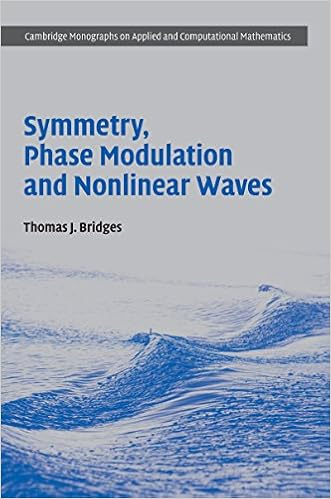Develops a natural approach to the problem based on phase modulation. It is both an elaboration of the use of phase modulation for the study of nonlinear waves and a compendium of background results in mathematics, such as Hamiltonian systems, symplectic geometry, conservation laws, Noether theory, Lagrangian field theory and analysis, all of which combine to generate the new theory of phase modulation.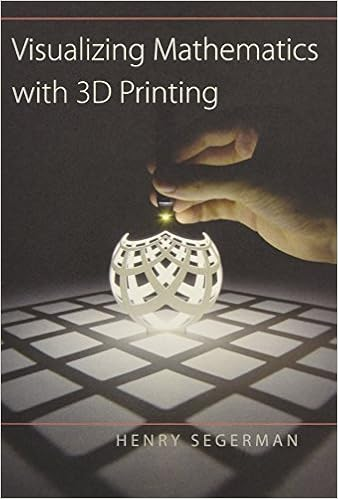Mathematician and mathematical artist Henry Segerman takes readers on a fascinating tour of two-, three-, and four-dimensional mathematics, exploring Euclidean and non-Euclidean geometries, symmetry, knots, tilings, and soap films.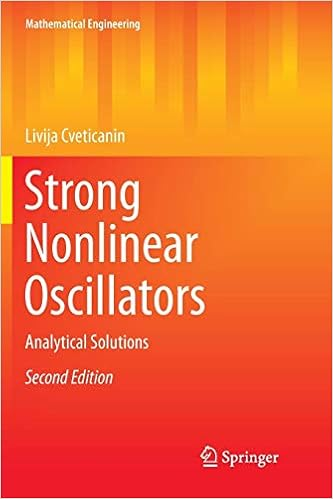In this second edition of the book, the number of approximate solving procedures for strong nonlinear oscillators is enlarged and a variety of procedures for solving free strong nonlinear oscillators is suggested. A method for error estimation is also given which is suitable to compare the exact and approximate solutions.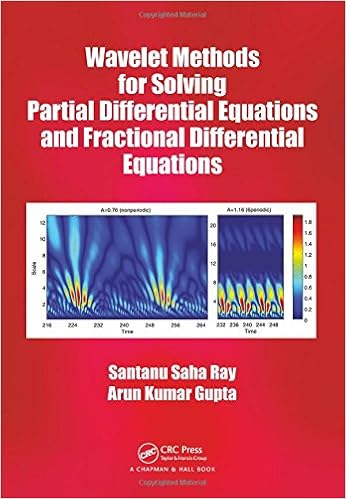The main focus of the book is to implement wavelet based transform methods for solving problems of fractional order partial differential equations arising in modelling real physical phenomena. It explores analytical and numerical approximate solution obtained by wavelet methods for both classical and fractional order partial differential equations.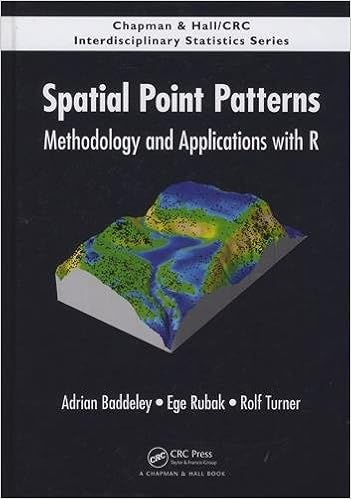Shows scientific researchers and applied statisticians from a wide range of fields how to analyze their spatial point pattern data.

## Welcome

This subject guide in Mathematics will assist you in your research needs as well as in learning about library resources.

## Technical Reference LibrarianJoseph J. Mercuri
Technical Reference Librarian
Van Houten Library, CAB 1040
973-596-8498
jmercuri@njit.edu

 New Jersey Institute of Technology University Heights, Newark, New Jersey 07102-1982 (973) 596-3206 Contact Us | Ask A Librarian |  Map & Directions | A to Z Site Index Copyrighted 2020 | Robert W. Van Houten Library